### Home > INT3 > Chapter Ch9 > Lesson 9.1.5 > Problem9-70

9-70.
1. Solve the following equations. Homework Help ✎

1.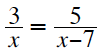2.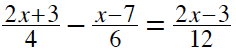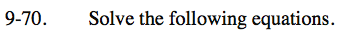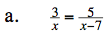Multiply both sides of the equation by the common denominator.

Simplify and combine like terms.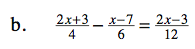Follow the same steps from part (a) and remember to distribute the negative sign.

x = −13